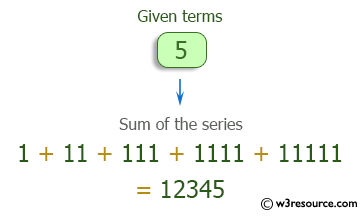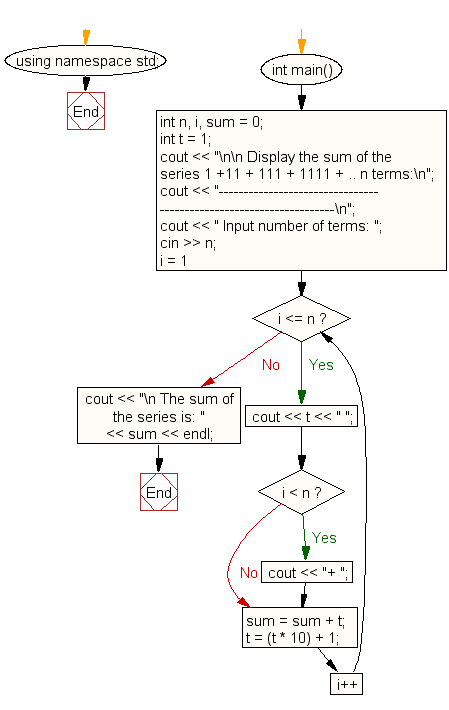﻿ C++ : Find the sum of the series 1+11+111+1111+ .. n terms

# C++ Exercises: Find the sum of the series 1 +11 + 111 + 1111 + .. n terms

## C++ For Loop: Exercise-26 with Solution

Write a program in C++ to find the sum of the series 1 +11 + 111 + 1111 + .. n terms.

Pictorial Presentation:Sample Solution:-

C++ Code :

``````#include <iostream>
using namespace std;

int main()
{
int n, i, sum = 0;
int t = 1;
cout << "\n\n Display the sum of the series 1 +11 + 111 + 1111 + .. n terms:\n";
cout << "-------------------------------------------------------------------\n";
cout << " Input number of terms: ";
cin >> n;
for (i = 1; i <= n; i++)
{
cout << t << " ";
if (i < n)
{
cout << "+ ";
}
sum = sum + t;
t = (t * 10) + 1;
}
cout << "\n The sum of the series is: " << sum << endl;
}
``````

Sample Output:

``` Display the sum of the series 1 +11 + 111 + 1111 + .. n terms:
-------------------------------------------------------------------
Input number of terms: 5
1 + 11 + 111 + 1111 + 11111
The sum of the series is: 12345
```

Flowchart:C++ Code Editor: